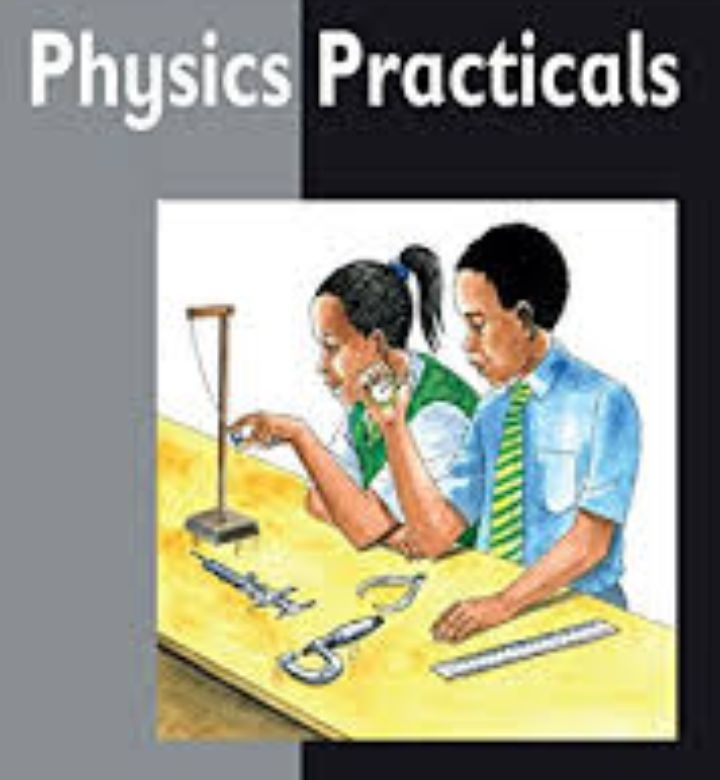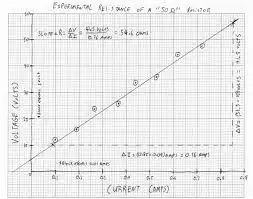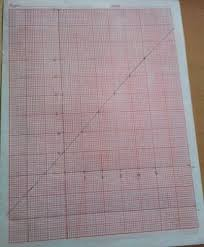Sign in# How to plot your Physics practical graph properly for SSCEPlotting a graph in your Physics practical is always occurent in external examinations for all candidates that are science students.

However, some students find particular arrears difficult in their practical, especially in their graph work, and these students may fail to get necessary knowledge of making a good practical work, which results to poor performance, even in SSCE.

In your Physics practical, the examiner marks your practical work mainly by the following sub-headings:

* Observations

* Graph

* Slope

* Deductions

* Accuracy

Below are important steps you should take when plotting your graph:

1. At the beginning of the drawing, start from the origin (0,0) if told to do so, and ensure you draw accurately a line for the y-axis and x-axis.

2. Any reading(s) taken from the apparatus used (e.g, meter rule, stop clock, ammeter, voltmeter, e.t.c) must be recorded to at least one decimal place, e.g, 0.250, 0.333, not 5, 3, 45, e.t.c.

3. Scales using multiple or sub- multiples of prime numbers, e.g, 3,7,9,13, e.t.c are not acceptable. Also, you should not forget to state the scale you used on top of the graph sheet, e.g, 2cm represent 10 volts at y-axis, 1cm represent 5 ohms at x-axis.

4. Do not make use of shady points on the graph.

5. Make use of a pencil first on your observation tables first before using a pen to avoid untidy writings. Failure to do so brings suspicion of cheating and leads to deductions of marks.

Line of best fit

This is the graduating line on your graph that is drawn where your reading points are placed.

To obtain a suitable line of best fit mark, at least three(3) points must be correctly plotted. Also, your line must be balanced, i.e, it should have points above and below the line and at both ends of the line.

The graph below shows an outstanding balance of reading points to the line, and the type of scale used:You should not force the line to pass through any specified data points, or try to alter results to get the line of best fit.

The purpose of the line of best fit is to reveal the trend of all data provided in the experiment, how y-axis varies proportionally with x-axis, and to find the gradient (slope) of the graph.

Slope

These is the measure of the change in y-axis to x-axis, i.e, dy/dx.

Slope is usually m as in equation of a straight line: y = mx + c

Slope/gradient = (Y2 - Y1) / (X2 - X1)

* Choose any two points far apart in y-axis and x-axis, and draw a right angle triangle that occupies at least one-third of your graph working.

* Note that the larger the triangle, the more accuracy of the slope. This does not mean an exaggerated size or magnitude of the triangle.

Below are detailed graph drawings of a slope:Intercept

It is a point where your line of best fit touches the y-axis (vertical) . Your question may state x-axis. The intercept should be given in at least one decimal place if necessary. In the graph (1) above, the intercept is 7.5 volts.

Precautions

The questions in practicals usually ask to state precautions to ensure accurate results. Below are certain basic precautions that you should consider:

1. Repeated readings: When a candidate repeats readings, they are trying to ensure that any errors that may have occurred in the readings are corrected. If such step is taking, it must be indicated in the table of values.

2. Parallax error: This is an apparent change in position of an object caused by change in position of the observer.

The anonymous precautions depends on the practical questions given. For example, in light/optics practical, we can include: Optical pins inserted vertically upright and spaced properly, Ensured the glass prism was well placed on rectangle drawn, e.t.c

Miscellaneous information

* Ensure that you indicate the units of all quantity given, e.g Voltage in volts, Length in cm, Time in seconds, e.t.c

* Always check the time allocated for the practical, so that you finish your working in time.

In conclusion, practice makes perfect. I recommend you practice drawing of physics graphs with already provided information or table of values, like the values provided in your past questions booklet.

I wish you good luck in your upcoming examinations.

Content created and supplied by: Timisilver (via Opera News )

Physics SSCE